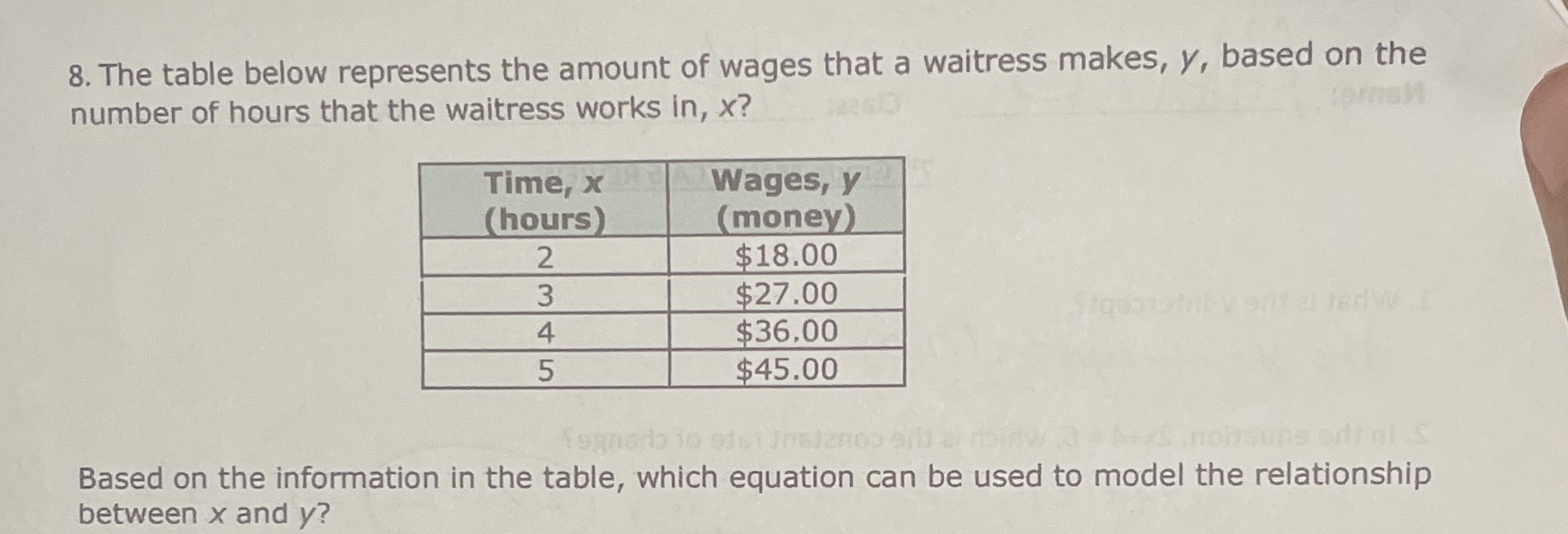### ¿Todavía tienes preguntas de matemáticas?

Pregunte a nuestros tutores expertos
Algebra
Pregunta8. The table below represents the amount of wages that a waitress makes, $$y$$ , based on the number of hours that the waitress works in, $$x$$ ?

Based on the information in the table, which equation can be used to model the relationship between $$x$$ and $$y$$ ?

$$y= 9x$$